### Home > GC > Chapter 1 > Lesson 1.2.5 > Problem1-96

1-96.

Copy the diagrams below on graph paper. Then find each result when each indicated transformation is performed.

1.   Reflect A across line $l$.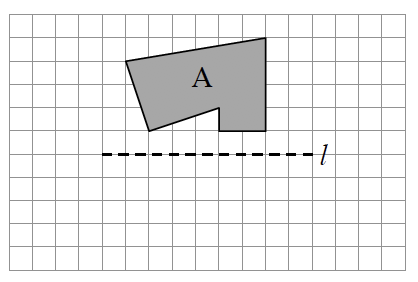1. Rotate B $90°$counterclockwise ($\circlearrowleft$) about point $P$.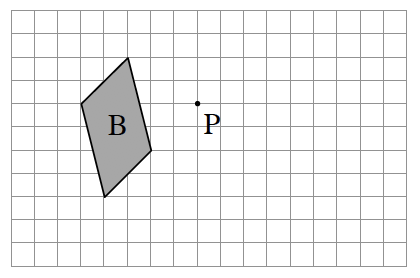c.   Rotate C $180°$ about point $Q$.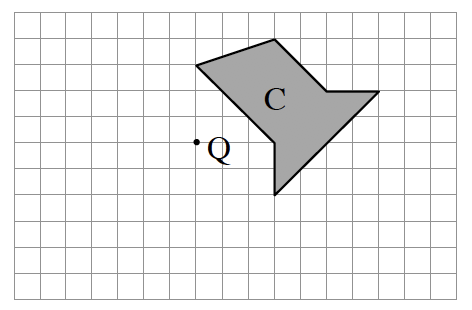1. Reflect D across line $m$.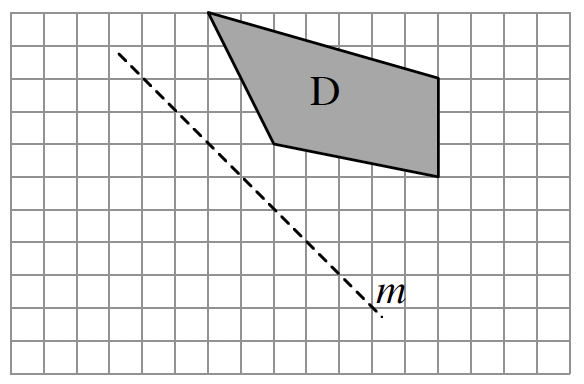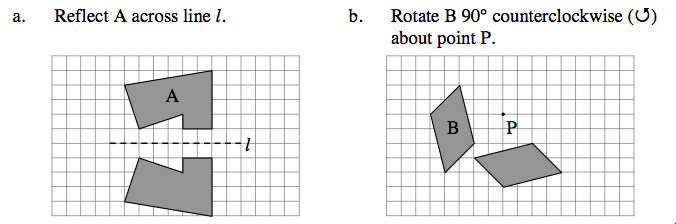See part (b) for help with part (c).

See part (a) for help with part (d).